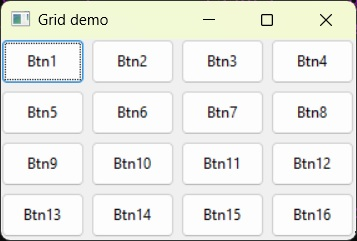# wxPython - GridSizer

As the name suggests, a GridSizer object presents a two dimensional grid. Controls are added in the grid slot in the left-to-right and top-to-bottom order. GridSizer object takes four parameters −

```wx.GridSizer(rows, columns, vgap, hgap)
```

vgap and hgap parameters control vertical and horizontal spacing between the adjacent controls.

Following table shows some important methods of wxGridSizer class −

S.N. Methods & Description
1

Adds a control in the next available grid slot

2

Adds each item in the list of controls

3

SetRows()

Sets the number of rows in the sizer

4

GetRows()

Retrieves the number of rows in the sizer

5

SetCols()

Sets the number of columns in the sizer

6

GetCols()

Retrieves the number of columns in size

7

SetVGap()

Sets vertical gap (in pixels) between the cells

8

GetVGap()

Returns the value of vgap between the cells

9

SetHGap()

Sets the horizontal gap (in pixels) between the cells

10

GetHGap()

Returns the value of hgap between the cells

The following code demonstrates a simple gridsizer of a 4 by 4 grid with vertical and horizontal gap of 5 pixels.

```Gs = wx.GridSizer(4, 4, 5, 5)
```

Sixteen button objects are successively added using a ‘for’ loop.

```for i in range(1,17):
btn = "Btn"+str(i)
```

The complete code is as follows −

```import wx

class Example(wx.Frame):

def __init__(self, parent, title):
super(Example, self).__init__(parent, title = title,size = (300,200))

self.InitUI()
self.Centre()
self.Show()

def InitUI(self):

p = wx.Panel(self)

gs = wx.GridSizer(4, 4, 5, 5)

for i in range(1,17):
btn = "Btn"+str(i)